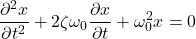# Damping Ratio

#### Engineering

In many engineering applications, damping ratio provides an important dimensionless parameter that describes the decay of oscillations in a system. From a system’s equilibrium, external disturbances may cause displacements in a system from which the system will oscillate. Commonly, spring-mass systems offer the best representation of the effects and necessity of damping ratio. Damping ratio is useful among many specific disciplines of engineering including controls, mechanical, and structural engineering. Damping ratio is also useful in the examination and applications of many different systems and large structures. This article will cover an in-depth definition of damping ratio, the formula and how it is useful, how it relates to natural frequency, and common applications.

## Damping Ratio Definition

In the analysis of mechanical systems, damping ratio plays a critical role in defining oscillatory motions. Damping ratio is a unitless parameter that helps define more quantitatively, the dissipation of energy. Oscillations are set off initially by disturbances that bring imbalances in a system. Disturbances to a system may come in the form of external forces, drag forces, nd even resistance in electrical applications. Symbolically speaking, damping ratio is denoted by the symbol zeta.The ranges of values for damping ratio help to mathematically express and identify the nature of the system in question. For the frequency response of a second-order system, meaning the system takes on characteristics associated with a second-order differential equation, the damping ratio is as follows from the second-order equation.## Damping Ratio and Natural Frequency

In the analysis of mechanical systems, natural frequency and damping ratio interact to help produce a more stable system. Natural frequency is the rate at which a system naturally vibrates or oscillates. Theoretically, harmonic oscillation depicts the natural frequency of an object as they are sinusoidal waves when displacement plots over time. In any system, excessive vibrations that match the natural frequency of system may cause structural and working failures and subsequently catastrophic damage. When a systems’ natural frequency matches its resonant frequency, the the sin waves peak become amplified. This amplification causes extreme fluctuations on a system. In designing any such mechanical or structural system it is important to ensure the system does not encounter excitations that match the natural frequency of the system.

This consideration is where damping ratio comes into play. Increasing natural frequency reduces the likelihood of an excitation frequencies’ ability to match. Alternatively, adding or even increasing damping a system will greatly diminish catastrophic excitations and deaden the response magnitude. Ideally, these two parameters working together are key to the proper design and safety considerations of many mechanical and structural systems and the loads that they under go.

### Different Levels of Damping

Recall the formula for damping ratio.Utilizing this formula, the degree of system damping may be identifiable. Of the different levels of damping, the four levels of damping are undamped, underdamped, overdamped, and critically damped. Each of these levels has characteristics of oscillation that are unique to their level of damping which is easily visible on graphs showing the system’s displacement over time.

#### Undamped

Undamped systems have a damping ratio of 0. These systems possess a characteristic sinusoidal shape to their displacement graph. Resulting graph shapes are because under ideal conditions and absent external forces, an undamped system oscillates infinitely. The undamped case shows the importance for systems to be damped in real-life cases as undamped harmonic motion may be catastrophic if left to oscillate.

#### Underdamped

Systems that have a damping ratio greater than 0 but less than 1 are underdamped. Underdamped systems have a characteristic sinusoidal shape to their displacement graph that will decay over time. Additionally, underdamped systems decay slower than all other cases besides undamped cases. Underdamped systems model how under damping may allow for some exaggerated oscillations that may negatively affect a real-life system.

#### Overdamped

A system that has a damping ratio greater than 1 is overdamped. Typically, overdamped systems have a characteristic flat shape to their displacement graph that decays quickly without the allowance of any sin waves. Overdamped systems comparatively are the flattest of the different cases. Similarly, the overdamped case shows how important it is for systems to be damped correctly in real-life cases. Overdamped systems may be modified to yield ideal design parameters in a system.

#### Critically Damped

Systems that have a ratio of 1 are critically damped. Critically damped systems have a characteristic flat shape to their displacement graph that decays quicker than an overdamped case in a more rounded way. Critically damped systems move the quickest towards equilibrium and without oscillating. The graph below accurately represents each of the cases on a displacement over time line graph.